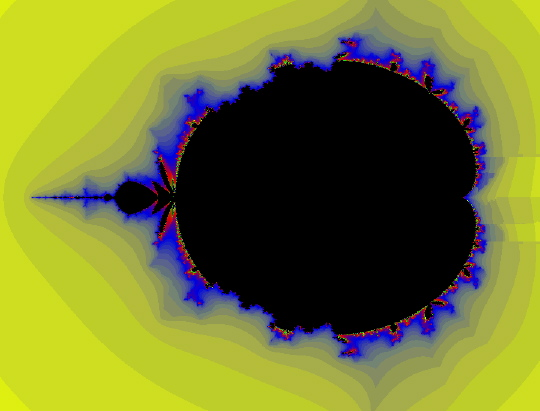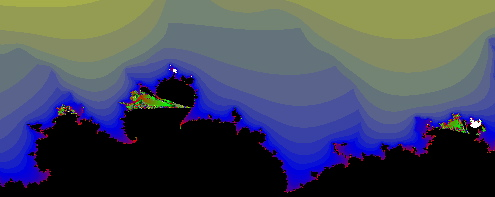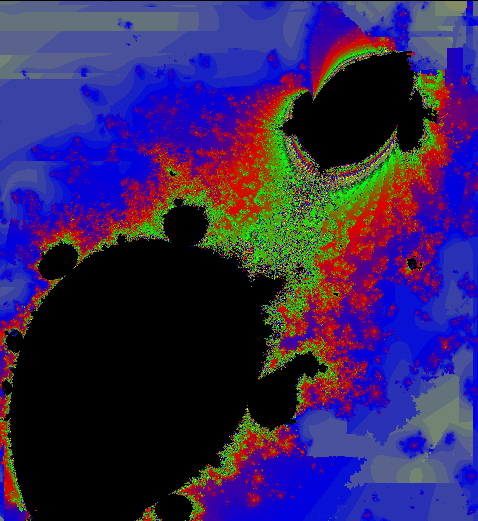Henon Map Parameter Space with SaddleDrop

The following images were all created using SaddleDrop.

Below: the c-plane for fixed a=0.15. Colored by fastest escaping critical point (connecteds).[-1.8125 <= Re(c) <= 0.7, -1 <= Im(c) <=0.9251]

Below is a zoom of the c-plane for fixed a=.3, near the analog of the rabbit bulb.
The coloration is by fastest escaping critical point (connecteds).[-0.63 <= Re(c) <= -0.166, 0.466 <= Im(c) <= 0.7466]

Below: a zoom of the c-plane for fixed a=.3, near the analog of the 1/5-bulb. Colored by fastest escaping critical point (connecteds).[0.04155 <= Re(c) <= 0.275, 0.4225 <= Im(c) <=0.275]

Below is something very strange looking. This is not a blowup, but a picture of nearly all of the black region for an a-plane [-.8 <= Re(a), Im(a) <= .8], with constant c=.3+.4i.
Here black rougly corresponds to maps whose Julia sets are not cantor sets. (coloration by slowest escaping critical point, horseshoes).